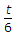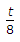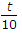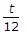# Civil Engineering - UPSC Civil Service Exam Questions

1.

The initial and final void ratios of a clay sample in a consolidation test are 1 and 0.5, respectively. If the initial thickness of the sample is 2.4 cm, then its final thickness will be

 A. 1.3 cm B. 1.8 cm C. 1.9 cm D. 2.2 cm

Explanation:

No answer description available for this question. Let us discuss.

2.

Cohesion is 15 kN/m2, the unit weight of soil is 20 kN/m3, the factor of safety is 1.5 and stability number is 0.05; the safe maximum height of the slope is

 A. 5.0 m B. 8.0 m C. 10.0 m D. 12.0 m

Explanation:

No answer description available for this question. Let us discuss.

3.

The nail diameter should not be more than(t = least thickness of the wooden member to be connected)

 A.B.C.D.Explanation:

No answer description available for this question. Let us discuss.

4.

As per practice of Indian Railways, the grade compensation provided for B.G. on curves is

 A. 0.5% degree B. 0.2% degree C. 0.4% degree D. 0.15% degree

Explanation:

No answer description available for this question. Let us discuss.

5.

In cement concrete pavements, tie bars are installed in

 A. expansion joints B. contraction joints C. warping joints D. longitudinal joints